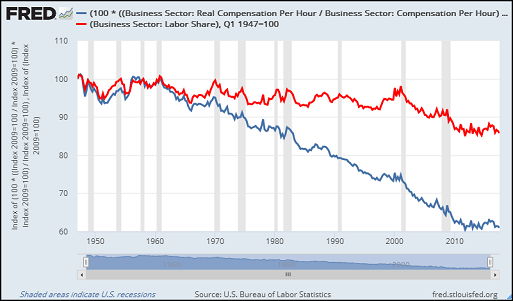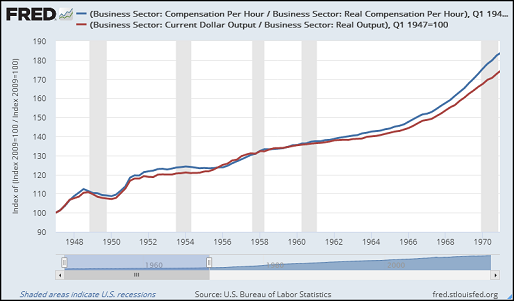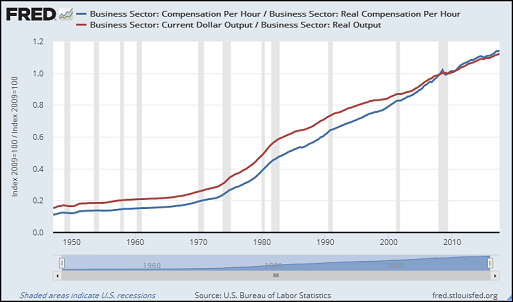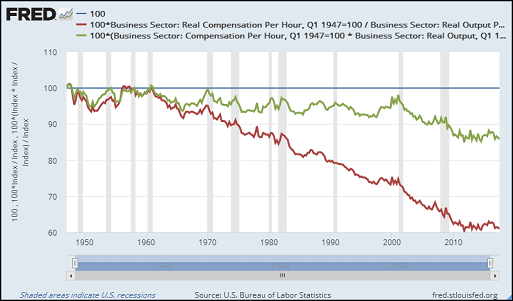## Friday, December 22, 2017

###Business Sector Price Indexes

This graph, from 18 December, shows real and nominal Labor Share. Does it remind you of anything?Graph #1: Labor Share, nominal (red) and real (blue), Indexed to 1947 Q1
Hint: It shows a gap that opens in 1961 and increases relentlessly in size thereafter.

You got it -- We see the same gap between productivity and compensation:Graph #2: Real Output per Hour (blue) and Real Compensation per Hour
Interesting. I wonder why that is.On Graph #1, the red line (labor share in the business sector) shows compensation relative to output. The blue line shows the same. The only difference is that the red line is a ratio of nominals and the blue is a ratio of reals.

If you have a knee-jerk reaction to this, it is probably that the ratio of nominals and the ratio of reals should be identical. I've seen it said about Debt-to-GDP, for example, that the ratio of nominals and the ratio of reals are identical.

Sometimes yes, sometimes no. For debt relative to GDP, the ratio of reals and the ratio of nominals are identical if you use the same calculation for debt as for GDP -- the calculation that converts nominal values to reals. But if you use one calculation to convert GDP to real values, and some other calculation to convert debt, you are almost guaranteed to get ratios that are not identical.

Something even simpler explains the difference visible on Graph #1: Real output and real compensation are figured using different price indexes. Here are the price indexes for Business Sector Output and Business Sector Compensation:Graph #3: Business Sector Price Indexes for Compensation ( blue) and Output (red) The Compensation Index is Higher than the Output Index, and Comes Down More when Deflated
I didn't know. I never saw them before. But the two price indexes had to be different: The arithmetic screams it. Why different indexes are used, I wouldn't know. You'd have to ask an economist.

On Graph #3 you can see a gap opening between the lines in the 1970s. And you might be able to see the upper edge of the blue line creeping out from under the red line some time around 1970. To get a better look at what is going on, I took Graph #3 and cut off everything after 1970, letting the rest expand:Graph #4: The Business Sector Price Indexes, 1947-1970
It is easy to see now that the gap between the red and blue lines goes all the way back to 1962. And again you can see the blue line creeping out from behind the red -- this time during the 1960-61 recession. Call it 1961.

That year sound familiar? 1961 is also the year that marks the start of the gap between real and nominal labor share on Graph #1 above. And 1961 marks the start of the gap between productivity and compensation on Graph #2.

All these graphs show a gap opening in 1961 and expanding relentlessly thereafter. I will come back to this thought shortly.For all these graphs, I indexed both lines on their start values so we can see how things change from the beginning. Economists don't seem to get that. If you don't index 'em on their start values, the two lines appear to run roughly parallel since 1947, and then the gap closes in 2009 -- because the source data is indexed on that date. Here's what Graph #3 looked like before I indexed the two lines on their start dates:Graph #5: Before I Indexed Graph #3 at 1947, It Looked Like This
The only difference between Graph #3 and Graph #5 is that in Graph #3, the two lines are pinned together in 1947 by indexing. In Graph #5, the two lines are pinned together in 2009 by default.

If we get rid of all the data before 2009 where the lines are pinned together, and then wait 70 years or so while more data is gathered and added to Graph #5, the graph will give you an idea of how the two price indexes have changed since 2009.

I get a similar effect on Graph #3 by pinning the lines together at 1947. We don't have to wait 70 years because the time has already gone by. We can just look at Graph #3 and get an idea how the two price indexes have changed since 1947.

The purpose of a price index is to show how much prices have gone up over a period of time. On Graph #5, both lines show that prices have gone up. But because the two lines are pinned together near the end, rather than at the beginning, the graph creates the false impression that there is little difference between the two lines. Pinning the lines together where they start -- as on Graph #3 -- is a way to correct this false impression.The gap on Graph #3 opens in 1961 and expands relentlessly thereafter, exactly like the gap on the first two graphs. Is this a coincidence?

It is not coincidence.

Graph #1 shows a gap between nominal labor share and real labor share. The only difference in the calculations is that real labor share uses the price indexes, and nominal does not. So it has to be the price indexes that create the gap on Graph #1.

Since there are no other differences in the calculation, it has to be the price indexes that create the gap. The fact that the labor share gap and the price index gap both start in 1961 is evidence that the price indexes create the gap in the labor share graph. The fact that both graphs show similar gap growth is additional evidence. That's evidence, not coincidence.

If I take the higher line on the labor share graph as a percent of the lower line, and do the same for the price index graph, I can put the two gaps together and compare them:Graph #6: Gap Size Comparison for Labor Share (blue) and the Price Indexes (red) Click the graph for a larger view
Identical. The gaps are identical.The story of the gap in the Productivity vs Compensation graph is not so neat and tidy. Even with nominal values there is a gap. Compensation per Hour and Output per Hour run more or less parallel, but are not identical:Graph #7: The Nominal Version of Productivity (blue) and Compensation (red) Note: Productivity (real output per hour) is a measure of economic growth, and economic growth is correctly measured in "real" rather than "nominal" values. This graph shows nominal "output per hour" values that will become "productivity" when the inflation is removed.
Compensation falls behind Output per Hour, even in the nominal data. When we bring price indexes into the calculation, the price index gap is combined with the gap in the nominals. The gap we so often see on graphs of Productivity vs Compensation is partly due to differences in the price indexes, and partly due to the differences between the nominals.

How much of the Productivity vs Compensation gap is due to price index differences? How much is due to differences between Output per Hour and Compensation per Hour, price indexes aside?

We can answer that question by using one price index instead of two. On the graph below, blue is productivity and red is compensation, figured as always, with the two different price indexes. The green line is compensation again, figured using the same price index that is used for the productivity numbers.

The gap between green and red is due to the price index. The gap between green and blue is due to compensation falling behind productivity.Graph #8: Productivity (blue), Compensation (red), and Compensation Deflated by the Same Price Index as Productivity (green)
If I take the red line as a percent of the blue line, we get a look at compensation relative to productivity, based on the way the two are always figured -- with different price indexes. I will show that in red on the next graph.

On the same graph I will show, in green, the green line as a percent of the blue, to see compensation relative to productivity when the two are figured with both using the same price index. This may give us a better measure of the gap between them.

I don't know yet if it's a better measure. I do know it's an issue. So I look.Graph #9: Two Measures of Compensation Falling Behind Productivity
The gap between the green and red lines on Graph #9 should remind you of the gaps on the first three graphs of this post. Graph #1 in particular is strikingly similar to #9.

The blue line on Graph #9 is the "goal" -- the level of Productivity, which is the level we expect Compensation to reach. Productivity is 100% of Productivity. Both measures of Compensation are generally less than 100% of Productivity, and show decline.

The red line is the compensation measure you would generally see on a "Productivity and Compensation" graph. The gap between blue and red shows how much Compensation has fallen behind. In the late 1950s, Compensation had not fallen behind at all. Since 2010, Compensation has been down to about 60% of Productivity; so, about 40% behind.

But the red Compensation uses a different measure of inflation than Productivity. The different measure may exaggerate the extent to which Compensation has fallen -- I'm not sure. (Whether it is "exaggeration" is a separate question.) The green Compensation line uses the same measure of inflation that is used for Productivity. Using the same price index for Compensation and Productivity eliminates this potential exaggeration.

The gap between blue and green shows how much Compensation has fallen behind without exaggeration. In the late 1950s, Compensation had not fallen behind at all. Since 2010, Compensation has been down to about 86% of Productivity. So, about 14% behind. About one-third of the gap.

But that's only if we figure it using the Output price index. If we figure it using the Compensation price index, Compensation is about 29% behind Productivity. Twice as much. Twice as far behind. Two-thirds of the gap.

But either way, Compensation per Hour is an average that does not count factors like rising inequality.

I'm gonna go take some aspirin now. Please feel free to check my work.I should add that at FRED, if I change the units for a data series to "Index (Scale value to 100 for chosen date)", the chosen date defaults to the data-start date 01/01/1947, which is exactly what I want.This series of posts begins on 14 December.

#### 1 comment:

The Arthurian said...

From BLS: Estimating the U.S. labor share

Footnote 7: "Although the output and compensation data used in calculating the labor share are not deflated, the output data used in estimating labor productivity and the compensation data used in estimating real hourly compensation are both deflated. The difference in these deflators makes up one component of the gap between real hourly compensation and labor productivity; the other component is the labor share."